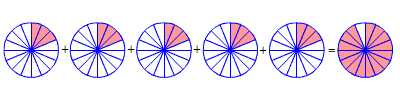# Multiplying a Fraction by a Whole Number

To multiply a fraction by a whole number, remember that multiplication is repeated addition.

Example 1:

Multiply $\frac{1}{7}\cdot 3$ .

Write the multiplication as an addition. Add $\frac{1}{7}$ three times.

$\frac{1}{7}\cdot 3=\frac{1}{7}+\frac{1}{7}+\frac{1}{7}$

Now we just need to add fractions with like denominators. Keep the denominators the same, and add the numerators.

$\begin{array}{l}=\frac{\left(1+1+1\right)}{7}\\ =\frac{3}{7}\end{array}$

Example 2:

Multiply $5\cdot \frac{3}{16}$ .

$\begin{array}{l}5\cdot \frac{3}{16}=\frac{3}{16}+\frac{3}{16}+\frac{3}{16}+\frac{3}{16}+\frac{3}{16}\\ =\frac{5\cdot 3}{16}\\ =\frac{15}{16}\end{array}$Another way to think about this is to rewrite the whole number as a fraction with the denominator of $1$ .

$5\cdot \frac{3}{16}=\frac{5}{1}\cdot \frac{3}{16}$

Then multiply numerators and denominators , as per the usual rules for multiplying fractions .

$\begin{array}{l}=\frac{5\cdot 3}{1\cdot 16}\\ =\frac{15}{16}\end{array}$

In some cases, your answer might be greater than $1$ , so you will want to rewrite it as a mixed number . You might also have to reduce the fraction to get it in simplest form.

Example 3:

Multiply $\frac{1}{4}\cdot 10$ .

$\frac{1}{4}\cdot 10=\frac{10}{4}$

Both the numerator and denominator have a common factor of $2$ . Divide both by $2$ .

$=\frac{5}{2}$

Rewrite this improper fraction as a mixed number.

$=2\frac{1}{2}$